Hoeffding decomposition

Letbe independent identically distributed random functions with values in a measurable space(cf. Random variable). For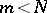, let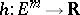be a measurable symmetric function invariables and consider the-statistics (cf.-statistic)The following theorem is called Hoeffding's decomposition theorem, and the representation of the-statistic as in the theorem is called the Hoeffding decomposition of(see [a1]):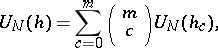where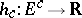is a symmetric function inarguments and where the-statisticsare degenerate, pairwise orthogonal in(uncorrelated) and satisfy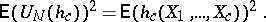The symmetric functions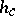are defined as follows: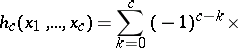Extensions of this decomposition are known for the multi-sample case [a4], under various "uncomplete" summation procedures in the definition of a-statistic, in some dependent situations and for non-identical distributions [a3]. There are also versions of the theorem for symmetric functions that have values in a Banach space.

The decomposition theorem permits one to easily calculate the variance of-statistics. Since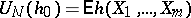and sinceis a sum of centred independent identically distributed random variables, the central limit theorem for non-degenerate-statistics is an immediate consequence of the Hoeffding decomposition (cf. also Central limit theorem).

The terminology goes back to [a2].